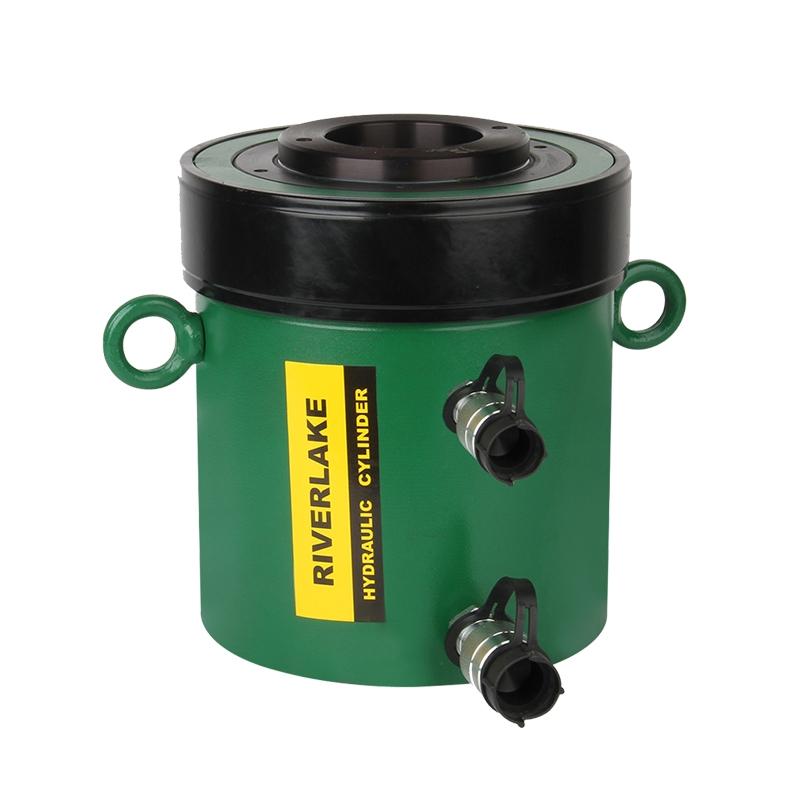December 8, 2023# How to Calculate Speed of Hydraulic Cylinder

If you’re looking for a guide on how to calculate the speed of a hydraulic cylinder, you’ve come to the right place. In this article, we’ll walk you through everything you need to know in order to make an accurate calculation. So whether you’re working on a project or just curious, keep reading for all the details!

## Hydraulic cylinders: what are they and how do they work?

A hydraulic cylinder is a device that uses compressed fluid to generate linear force. The fluid is typically incompressible, which means that it can only be displaced, not compressed. When the piston inside the cylinder is forced downward, the fluid is displaced and flows into the chambers on either side of the piston. This in turn creates pressure, which pushes against the piston and forces it upward.

The amount of force generated by the cylinder can be controlled by regulating the amount of fluid that is displaced. As a result, hydraulic cylinders are often used in devices that require precise control, such as robotics and construction equipment.

A double acting hollow hydraulic cylinder is a type of hydraulic cylinder that uses two distinct chambers, one on each side of a central piston, to create linear force. This design allows for greater force output than a single acting cylinder of the same size, as well as the ability to generate force in both directions.

## How to calculate the speed of a hydraulic cylinder?

There are many different ways to calculate the speed of a hydraulic cylinder, but all of them involve some basic principles. The first thing you need to know is the volume of fluid that the cylinder is designed to hold. This can be found in the product manual or on the cylinder itself. Once you have this number, you need to determine the rate at which fluid is being added to or subtracted from the cylinder. In most cases, this is measured in liters per minute.

Finally, you need to know the diameter of the piston inside the cylinder. With all of this information, you can use the following formula to calculate the speed of the piston: Speed (in meters per second) = (Fluid flow rate (in liters per minute)) / ((π*(Piston diameter (in meters))^2)/4). With this information, you can easily calculate the speed of any hydraulic cylinder.

## The factors affecting the speed of a hydraulic cylinder

Hydraulic cylinders are used in a wide variety of applications, from construction and manufacturing to automotive and aerospace. The speed at which a hydraulic cylinder can operate is determined by a number of factors, including the size of the cylinder, the type of fluid used, and the pressure of the system. The size of the cylinder affects the speed because it determines the amount of fluid that can be displaced in a given amount of time.

The type of fluid used also affects the speed, as different fluids have different viscosities. Finally, the pressure of the system plays a role because it determines how much force is required to move the pistons in the cylinder. By understanding these factors, engineers can optimize hydraulic systems for specific applications.

## Final Thoughts

In conclusion, to calculate the speed of a hydraulic cylinder we need to know its bore, stroke, and rod diameter. We can use this information to determine the volume of fluid displaced with each movement of the piston and then calculate how much time it takes for that volume displacement to occur. This calculation gives us the speed of the hydraulic cylinder in terms of feet per minute or meters per second.